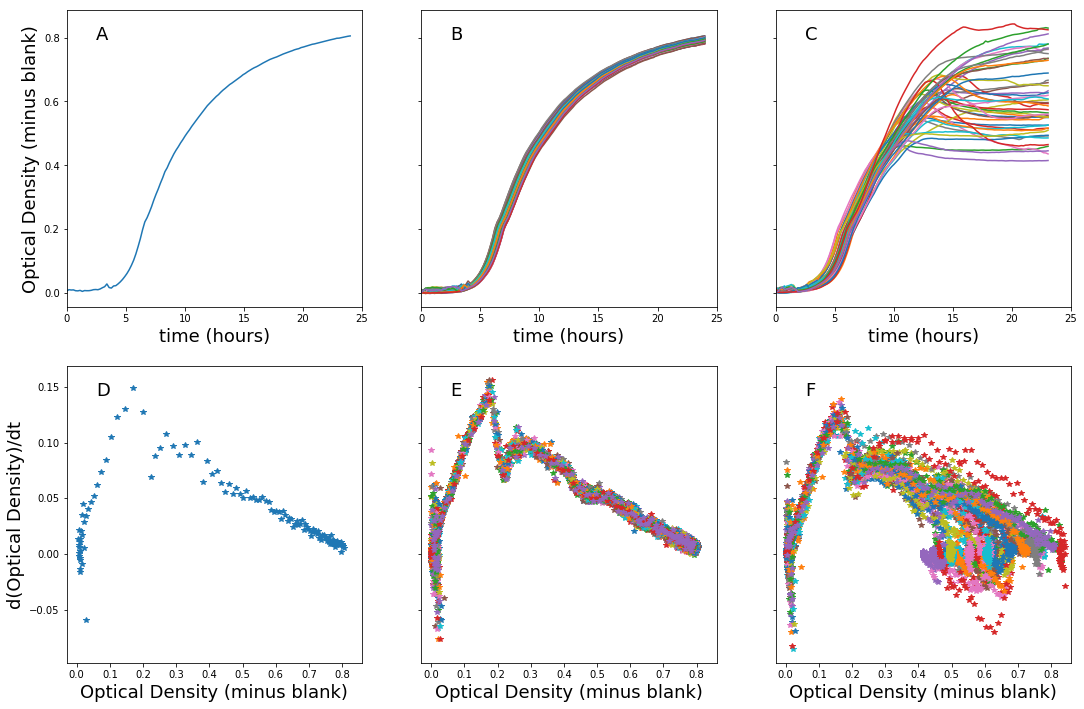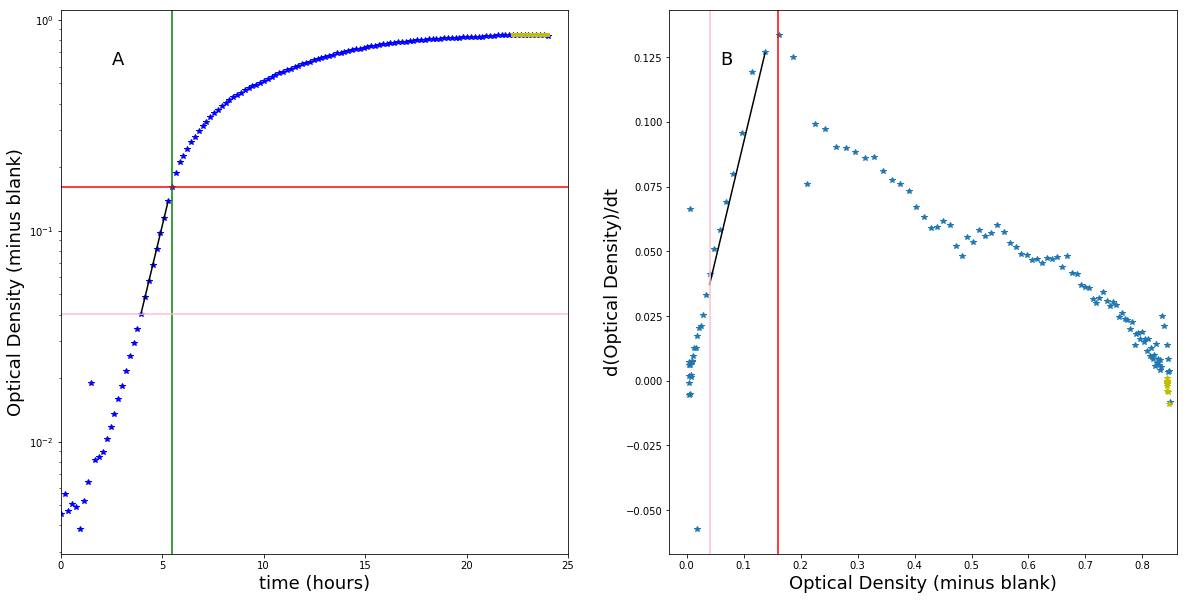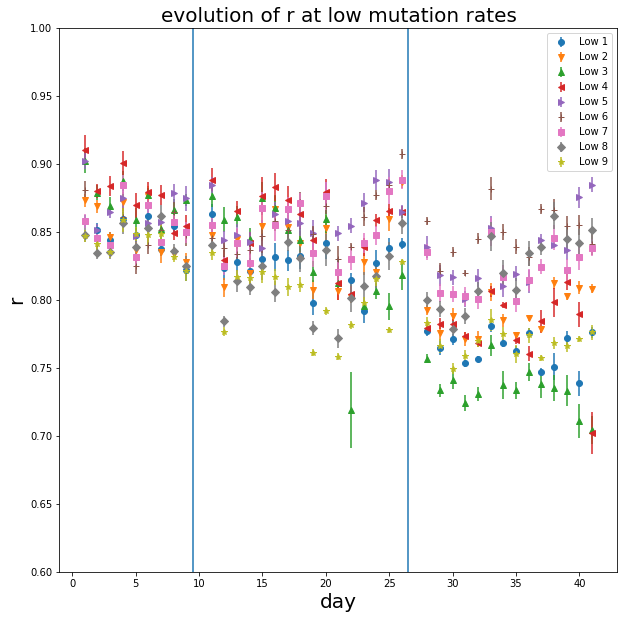PK \sAU�� Estimating NS001 per OD600.htmlUT 0N8c0N8c Estimating NS001 per OD600
In :
import numpy as np

In :
# Conversion factor from CFU on plate to CFU in well, and daily dilution factor during evolution experiment

CFUS_PER_ML_OVER_PLATE = 10**7
DAILY_DILUTION = 500

# Ancestral CFU counts and OD600 in platereader after growing at 30 degrees Celsius
Ancestral_NS001_Plating_Counts = np.array([54, 28, 15, 36, 24, 20, 33, 40, 11, 61, 47, 22, 45,
46, 74, 19, 37, 20, 35, 20, 23, 48, 13, 17, 16, 16,
17, 18, 45, 47, 81, 34, 51, 32, 50, 60, 78, 64, 45,
18, 20, 72, 68])
Ancestral_NS001_OD600s = np.array([0.276, 0.28613, 0.26408, 0.2913, 0.25755, 0.29297, 0.231,
0.16795, 0.16625, 0.20347, 0.19645, 0.15123, 0.18605, 0.2149,
0.15932, 0.18525, 0.15868, 0.18548, 0.15317, 0.16133, 0.18188,
0.17113, 0.1333, 0.14218, 0.16023, 0.18067, 0.17688, 0.2094,
0.21363, 0.18075, 0.1815, 0.14425, 0.15232, 0.17988, 0.17058,
0.16795, 0.15892, 0.17345, 0.13955, 0.15837, 0.14715, 0.14608,
0.1641])
Ancestral_NS001_OD_Blanks = np.array([.0794, .0791, .0869])

# Final Strain CFU counts and OD600 in platereader after growing at 30 degrees Celsius

High1_Plating_Counts = np.array([109, 128, 116, 113, 125, 178, 118, 117])
High1_OD600s = np.array([0.31783, 0.30065, 0.30998, 0.28337,
0.31538, 0.333, 0.29463, 0.28297])

HiMid1_Plating_Counts = np.array([136, 126, 203, 174, 225, 130, 223, 128, 144])
HiMid1_OD600s = np.array([0.38885, 0.36762, 0.37105, 0.38145, 0.3728,
0.4085, 0.38412, 0.3699, 0.35283])

Mid1_Plating_Counts = np.array([159, 143, 128, 186, 103, 133, 112, 105])
Mid1_OD600s = np.array([0.26927, 0.28328, 0.29468, 0.27982, 0.28565,
0.28475, 0.27565, 0.24793])

LoMid1_Plating_Counts = np.array([125, 100, 125, 86, 129, 91, 111, 95])
LoMid1_OD600s = np.array([0.30528, 0.31983, 0.32877, 0.33193, 0.33168,
0.31457, 0.32398, 0.3037])

LoMid5_Plating_Counts = np.array([94, 88, 76, 114, 98, 91, 125, 72, 76])
LoMid5_OD600s = np.array([0.34545, 0.33127, 0.32858, 0.37605, 0.34335,
0.35247, 0.37815, 0.3294, 0.32993])

Low1_Plating_Counts = np.array([86, 109, 111, 127, 99, 58, 61])
Low1_OD600s = np.array([0.30783, 0.31777, 0.32932, 0.34125,
0.33075, 0.30223, 0.3058])

Low5_Plating_Counts = np.array([58, 74, 54, 55, 100, 56, 43, 52])
Low5_OD600s = np.array([0.1811, 0.23818, 0.21805, 0.21475, 0.225,
0.1919, 0.1701, 0.19787])

High_to_Mid_OD_Blanks = np.array([0.08205, 0.0804, 0.0784])
LoMid_to_Low_OD_Blanks = np.array([0.0835, 0.08365, 0.081225])

In :
Ancestral_CFU_per_ml_OD600 = (Ancestral_NS001_Plating_Counts
/ (Ancestral_NS001_OD600s - np.mean(Ancestral_NS001_OD_Blanks))
* CFUS_PER_ML_OVER_PLATE)

High1_CFU_per_ml_OD600 = (High1_Plating_Counts
/ (High1_OD600s - np.mean(High_to_Mid_OD_Blanks))
* CFUS_PER_ML_OVER_PLATE)

HiMid1_CFU_per_ml_OD600 = (HiMid1_Plating_Counts
/ (HiMid1_OD600s - np.mean(High_to_Mid_OD_Blanks))
* CFUS_PER_ML_OVER_PLATE)

Mid1_CFU_per_ml_OD600 = (Mid1_Plating_Counts
/ (Mid1_OD600s - np.mean(High_to_Mid_OD_Blanks))
* CFUS_PER_ML_OVER_PLATE)

LoMid1_CFU_per_ml_OD600 = (LoMid1_Plating_Counts
/ (LoMid1_OD600s - np.mean(LoMid_to_Low_OD_Blanks))
* CFUS_PER_ML_OVER_PLATE)

LoMid5_CFU_per_ml_OD600 = (LoMid5_Plating_Counts
/ (LoMid5_OD600s - np.mean(LoMid_to_Low_OD_Blanks))
* CFUS_PER_ML_OVER_PLATE)

Low1_CF_per_ml_OD600 = (Low1_Plating_Counts
/ (Low1_OD600s - np.mean(LoMid_to_Low_OD_Blanks))
* CFUS_PER_ML_OVER_PLATE)

Low5_CF_per_ml_OD600 = (Low5_Plating_Counts
/ (Low5_OD600s - np.mean(LoMid_to_Low_OD_Blanks))
* CFUS_PER_ML_OVER_PLATE)

In :
Ancestral_mean = np.mean(Ancestral_CFU_per_ml_OD600)
Ancestral_std = np.std(Ancestral_CFU_per_ml_OD600)

High1_mean = np.mean(High1_CFU_per_ml_OD600)
High1_std = np.std(High1_CFU_per_ml_OD600)

HiMid1_mean = np.mean(HiMid1_CFU_per_ml_OD600)
HiMid1_std = np.std(HiMid1_CFU_per_ml_OD600)

Mid1_mean = np.mean(Mid1_CFU_per_ml_OD600)
Mid1_std = np.std(Mid1_CFU_per_ml_OD600)

LoMid1_mean = np.mean(LoMid1_CFU_per_ml_OD600)
LoMid1_std = np.std(LoMid1_CFU_per_ml_OD600)

LoMid5_mean = np.mean(LoMid5_CFU_per_ml_OD600)
LoMid5_std = np.std(LoMid5_CFU_per_ml_OD600)

Low1_mean = np.mean(Low1_CF_per_ml_OD600)
Low1_std = np.std(Low1_CF_per_ml_OD600)

Low5_mean = np.mean(Low5_CF_per_ml_OD600)
Low5_std = np.std(Low5_CF_per_ml_OD600)

print("Final CFUs per ml of OD600 culture are estimated at")
print(f"Ancestral: {Ancestral_mean:.2E} +- {Ancestral_std:.2E}")
print(f"High1: {High1_mean:.2E} +- {High1_std:.2E}")
print(f"HiMid1: {HiMid1_mean:.2E} +- {HiMid1_std:.2E}")
print(f"Mid1: {Mid1_mean:.2E} +- {Mid1_std:.2E}")
print(f"LoMid1: {LoMid1_mean:.2E} +- {LoMid1_std:.2E}")
print(f"LoMid5: {LoMid5_mean:.2E} +- {LoMid5_std:.2E}")
print(f"Low1: {Low1_mean:.2E} +- {Low1_std:.2E}")
print(f"Low5: {Low5_mean:.2E} +- {Low5_std:.2E}")

Final CFUs per ml of OD600 culture are estimated at
Ancestral: 4.12E+09 +- 2.65E+09
High1: 5.58E+09 +- 6.68E+08
HiMid1: 5.58E+09 +- 1.35E+09
Mid1: 6.78E+09 +- 1.34E+09
LoMid1: 4.55E+09 +- 6.70E+08
LoMid5: 3.50E+09 +- 4.03E+08
Low1: 3.89E+09 +- 8.36E+08
Low5: 5.05E+09 +- 9.31E+08

In :
TRANSFER_VOLUME = .001
LOWEST_OD600 = .4
HIGHEST_OD600 = .9

Lowest_CFU_at_day_start_estimate = TRANSFER_VOLUME*LOWEST_OD600*(LoMid5_mean - LoMid5_std)
Highest_CFU_at_day_start_estimate = TRANSFER_VOLUME*HIGHEST_OD600*(Mid1_mean + Mid1_std)

print(f"The number of live cells at the start of each day would be"
f" between roughly {Lowest_CFU_at_day_start_estimate:.2E} and {Highest_CFU_at_day_start_estimate:.2E}.")

The number of live cells at the start of each day would be between roughly 1.24E+06 and 7.31E+06.

In [ ]:


PK�R����PK \sAU��*��* Evolution-Experiments.htmlUT 0N8c0N8c Description of the evolution experiments-Using simpler measure of saturating OD - Updated
In :
from collections import OrderedDict
import copy
import numpy as np
import matplotlib.pyplot as plt
import os
import scipy.optimize as spopt

%matplotlib inline


# Description of the Evolution Experiment¶

The evolution experiment (short compared to Lenski) was similar to Lenski's experiment. I started with a single strain of E. coli and split it into 45 populations that each grew in 500 microliters of supplemented M9 medium at 30 degrees Celsius in a platereader each day. There were also 3 blank wells. Over the course of the day, the E. coli consume their food and the platereader measures the optical density of the E. coli every ~10 minutes. At the end of each day, all 45 populations are diluted 500x and put into a fresh well of 500 microliters then back in the platereader.

Every three days, a sample of all populations is frozen in case of mishap. Twice the experiment was stopped, all stocks were frozen, and then the experiment was resumed later. Let's look at the growth curve data.

What makes this experiment different from Lenski's is that the strain of E. coli used has an adjustable mutation rate tuned by otherwise innocuous chemical inducers. 5 combinations of inducer concentrations were chosen giving 5 mutation rates with 9 replicates of each. Thus, for an otherwise fixed strain of E. coli we can see how the mutation rate affects evolution.

First I need to import the data.

In :
os.chdir("Experiment 1")

days = OrderedDict()

filename_end = ' July 2018 NS001 delta CAT 30 C.xlsx'
platelayout1 = ['High '+str(i) for i in range(1,10)] + ['HiMid '+str(i) for i in range(1,10)] + \
['Mid '+str(i) for i in range(1,10)] + ['LoMid '+str(i) for i in range(1,10)] + \
['Low '+str(i) for i in range(1,10)] + ['blank1', 'blank2', 'blank3']

for i in range(9,10):
filename = str(i) + filename_end
platelayout180 = copy.copy(platelayout1)
platelayout180.reverse() # I accidentally rotated the plate 180 degrees this day
days[i-9] = prdl.import_platereader(filename, platelayout180)

for i in range(10,19):
filename = str(i) + filename_end

os.chdir("../Experiment 1 Branch off July 18")

filename_end_branch = ' 2018 NS001 delta CAT mutation rate titrations.xlsx'

platelayout_branch = platelayout1

treatments = copy.deepcopy(platelayout_branch)
treatments.remove('blank1')
treatments.remove('blank2')
treatments.remove('blank3')

for i in range(0,8):
filename = str(i+24) + ' August' + filename_end_branch

for i in range(8,17):
filename = str(i-7) + ' September' + filename_end_branch

os.chdir("../Experiment 1 Branch 1 Continued 28 Sept")

filename_end_continue = ' 2018 NS001 delta CAT mutation rate titrations.xlsx'

for i in range(0,4):
filename = str(i+27) + ' September' + filename_end_branch

for i in range(4,15):
filename = str(i-3) + ' October' + filename_end_branch
days[i+27] = prdl.import_platereader(filename, platelayout_branch)


The days are numbered 0 through 41. The replicates are named by relative mutation rate and replicate number. We call them High, HiMid, Mid, LoMid, and Low.

From lowest to highest mutation rate the corresponding inducer conditions of IPTG (micromolar) and aTc (ng/mL) are (2000,10), (2000,2), (2000,0), (50,0), and (0,0).

What do these curves typically look like? Let's see Day 1 and Day 41. We'll estimate the blank from the median of all blanks across all days. Earlier analysis showed a fairly consistent blank well from day to day, so to avoid overfitting, we'll use the same level of blank every day.

# Growth curves on Day 1 vs Day 41¶

In :
def estimate_blank(data):
blanks = []
for key in data:
b1 = np.mean(data[key]['well_ODs']['blank1'])
b2 = np.mean(data[key]['well_ODs']['blank2'])
b3 = np.mean(data[key]['well_ODs']['blank3'])
blanks.append(np.median(np.array([b1,b2,b3])))
return np.median(np.array(blanks))

fig, axes = plt.subplots(2, 3, sharey='row', figsize=(18,12))
t = days['times']
P = days['well_ODs']['High 1'] - estimate_blank(days)
dP_dt = np.diff(P)/np.diff(t)
axes[0,0].plot(t, P);
axes[0,0].set_xlim([0,25]);
axes[0,0].set_xlabel('time (hours)', fontsize=18);
axes[0,0].set_ylabel('Optical Density (minus blank)', fontsize=18);
axes[0,0].text(.1, .9, 'A', transform=axes[0,0].transAxes, fontsize=18);
axes[1,0].plot(P[:-1], dP_dt, '*');
axes[1,0].set_xlim([-.03,.86]);
axes[1,0].set_xlabel('Optical Density (minus blank)', fontsize=18);
axes[1,0].set_ylabel('d(Optical Density)/dt', fontsize=18);
axes[1,0].text(.1, .9, 'D', transform=axes[1,0].transAxes, fontsize=18);

for sample in treatments:
t = days['times']
P = days['well_ODs'][sample] - estimate_blank(days)
dP_dt = np.diff(P)/np.diff(t)
axes[0,1].plot(t, P);
axes[1,1].plot(P[:-1], dP_dt, '*');
axes[0,1].set_xlabel('time (hours)', fontsize=18);
axes[0,1].set_xlim([0,25]);
axes[0,1].text(.1, .9, 'B', transform=axes[0,1].transAxes, fontsize=18);
axes[1,1].set_xlim([-.03,.86]);
axes[1,1].set_xlabel('Optical Density (minus blank)', fontsize=18);
axes[1,1].text(.1, .9, 'E', transform=axes[1,1].transAxes, fontsize=18);

for sample in treatments:
t = days['times']
P = days['well_ODs'][sample] - estimate_blank(days)
dP_dt = np.diff(P)/np.diff(t)
axes[0,2].plot(t, P);
axes[1,2].plot(P[:-1], dP_dt, '*');
axes[0,2].set_xlabel('time (hours)', fontsize=18);
axes[0,2].set_xlim([0,25]);
axes[0,2].text(.1, .9, 'C', transform=axes[0,2].transAxes, fontsize=18);
axes[1,2].set_xlim([-.03,.86]);
axes[1,2].set_xlabel('Optical Density (minus blank)', fontsize=18);
axes[1,2].text(.1, .9, 'F', transform=axes[1,2].transAxes, fontsize=18);# Extracting parameters describing the growth curves each day¶

There's clearly a great deal of regularity in the growth curves. An initial period where the signal to noise is too poor to get a good measurement, followed by exponential growth. Then a more complicated transition region. Then what looks like an exponential decay towards a saturating optical density.

So I'll analyze the data by extracting time to leave exponential, saturating optical density, and a curve fit for exponential phase

In :
def exp(t, P0, r):
return P0*np.exp(r*t)

def fit_exp(t, P, cut_below, cut_above):
try:
i1 = np.where(P<cut_below)[-1]+1
except IndexError:
i1 = 0
i2 = np.where(P<cut_above)[-1]+1
try:
popt_exp, pcov_exp = spopt.curve_fit(exp, t[i1:i2], P[i1:i2], p0=(.002, 1))
except RuntimeError:
popt_exp = np.ones(3)*np.nan
pcov_exp = np.ones((3,3))*np.nan
return popt_exp, pcov_exp

def time_to_leave_exp(t, P, cut_above):
i1 = np.where(P<cut_above)[-1]
i2 = i1+1
t1 = t[i1]
t2 = t[i2]
p1 = P[i1]
p2 = P[i2]
return t1 + (cut_above - p1)/(p2-p1)*(t2-t1)

def fit_over_experiments(data, days, samples, cut_below=.12, cut_above=.24):
# set up the variables that will be returned with parameter estimates
popt_exp = OrderedDict()
pcov_exp = OrderedDict()
K_est = OrderedDict()
t_cut2 = OrderedDict()
# estimate OD of empty well (blank) and adjust cutoffs by subtracting value of blank
blank = estimate_blank(data)
cut_below = cut_below - blank
cut_above = cut_above - blank
# estimate the parameters of exponential phase and the saturating OD
for sample in samples:
popt_exp[sample]=np.zeros((2,days))
pcov_exp[sample]=np.zeros((2,2,days))
K_est[sample]=np.zeros(days)
t_cut2[sample]=np.zeros(days)
for i in range(days):
t = data[i]['times']
P = data[i]['well_ODs'][sample] - blank
pexp, covexp = fit_exp(t, P, cut_below, cut_above)
popt_exp[sample][:,i] = pexp
pcov_exp[sample][:,:,i] = covexp
K_est[sample][i] = np.mean(P[-10:])
t_cut2[sample][i] = time_to_leave_exp(t, P, cut_above)
return popt_exp, pcov_exp, K_est, t_cut2, blank

In :
pexps, covexps, Ks, t_trans, blank = fit_over_experiments(days, 42, treatments)


What do these curve fits typically look like? A quick plot helps visualize how I'm extracting parameters.

In :
fig, axes = plt.subplots(1, 2, figsize=(20,10))
dy = 32
smp = 'HiMid 1'
t = days[dy]['times']
P = days[dy]['well_ODs'][smp] - estimate_blank(days)
i1 = np.where(P<.12-estimate_blank(days))[-1]+1
i2 = np.where(P<.24-estimate_blank(days))[-1]+1
dP_dt = np.diff(P)/np.diff(t)
exp_phase = pexps[smp][dy]*np.exp(pexps[smp][dy]*t[i1:i2])
sat_OD = Ks[smp][dy]*np.ones(10)

axes.semilogy(t, P, 'b*');
axes.set_xlim([0,25]);
axes.set_xlabel('time (hours)', fontsize=18);
axes.set_ylabel('Optical Density (minus blank)', fontsize=18);
axes.text(.1, .9, 'A', transform=axes.transAxes, fontsize=18);
axes.axvline(t_trans[smp][dy],color='green');
axes.axhline(.12-estimate_blank(days), color='pink');
axes.axhline(.24-estimate_blank(days),color='red');
axes.semilogy(t[i1:i2], exp_phase, 'k');
axes.semilogy(t[-10:], sat_OD, 'y', linewidth=4);

axes.plot(P[:-10], dP_dt[:-9], '*');
axes.plot(P[-10:-1], dP_dt[-9:], 'y*');
axes.plot(P[i1:i2], pexps[smp][dy]*P[i1:i2],'k');
axes.set_xlim([-.03,.86]);
axes.set_xlabel('Optical Density (minus blank)', fontsize=18);
axes.set_ylabel('d(Optical Density)/dt', fontsize=18);
axes.text(.1, .9, 'B', transform=axes.transAxes, fontsize=18);
axes.axvline(.12-estimate_blank(days), color='pink');
axes.axvline(.24-estimate_blank(days),color='red');Now I can plot the evolution of the exponential growth rate of the population over time, the evolution of the time to leave exponential, and the evolution of the saturating OD. Because there are 45 replicates, I show the data by mutation rate group. I plot slashes on the x-axis on the days where the experiment was interrupted by freezing and unfreezing. I also drop data from the first day, and from each day after an unfreezing because the initial population was not the same on these days as on other days and it affects the resulting parameters of the growth curve.

In :
days_s = [i for i in range(1,42) if i != 27 and i !=10] # days that aren't the first day or after waking up from freezing
markers = ['o', 'v', '^', '<', '>', '+', 's', 'D', '*']
colors = ['C0', 'C1', 'C2', 'C3', 'C4', 'C5', 'C6', 'C7', 'C8']


## Evolution of the growth rate¶

It turns out that the growth rate does not evolve much over time. At least not in a way discernible from noise. Although some curves seem to show small decreases in their exponential phase growth over time.

In :
plt.figure(figsize=(10,10))
param = 1
for i, sample in enumerate(['Low '+str(i) for i in range(1,10)]):
plt.errorbar(np.arange(42)[days_s], pexps[sample][param][days_s], yerr=covexps[sample][param,param][days_s]**.5,
linestyle='', marker=markers[i], color=colors[i], label=sample)
plt.title('evolution of r at low mutation rates',fontsize=20);
plt.xlabel('day', fontsize=20);
plt.ylabel('r', fontsize=20);
plt.axvline(x=9.5);
plt.axvline(x=26.5);
plt.legend()
plt.ylim(.6,1.0);In :
plt.figure(figsize=(10,10))
param = 1
for i, sample in enumerate(['LoMid '+str(i) for i in range(1,10)]):
plt.errorbar(np.arange(42)[days_s], pexps[sample][param][days_s], yerr=covexps[sample][param,param][days_s]**.5,
linestyle='', marker=markers[i], color=colors[i], label=sample)
plt.title('evolution of r at lomid mutation rates',fontsize=20);
plt.xlabel('day', fontsize=20);
plt.ylabel('r', fontsize=20);
plt.axvline(x=9.5);
plt.axvline(x=26.5);
plt.legend()
plt.ylim(.6,1.0);